#Function Repository Resource:

# CombinatorStep

Perform an update to a combinator expression based on defined rules and traversal scheme

Contributed by: Wolfram Research
 ResourceFunction["CombinatorStep"][cmb] performs a single leftmost outermost update on the combinator expression cmb with S and K combinator transformations. ResourceFunction["CombinatorStep"][cmb, scheme] performs an update on cmb according to matches found in scheme. ResourceFunction["CombinatorStep"][rules, cmb, scheme] performs update with combinator transformations specified by rules.

## Details and Options

The input cmb must be given as a combinator expression in nested bracket form.
If scheme is specified, direction ("Leftmost", "Rightmost") and depth order ("Outermost", "Innermost") must be specified, in order of their priority as sorting criteria.
scheme, which is a list, can include an optional third element, specified as an integer number or Infinity, that indicates how many matches should be applied in the update step.
The combinator transformations of interest should be given as a list of Rule or RuleDelayed elements in rules.
Automatic can be specified for either or both of rules and scheme.
ResourceFunction["CombinatorStep"] can take the following option:
 "SKGlyphs" symbols used to specify default transformation rules

## Examples

### Basic Examples (5)

Perform the default single leftmost outermost update on a combinator expression:

 In:=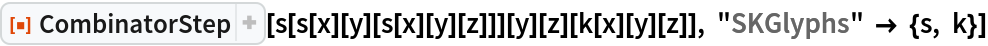Out=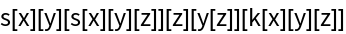In:=Out=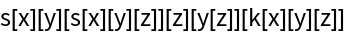Update a combinator with the first three matches found from the rightmost outermost order:

 In:=Out=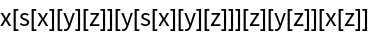In:=Out=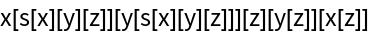Update an expression using only the K combinator transformation rule:

 In:=Out=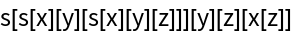Update an expression using only the S combinator transformation rule in a single-update leftmost outermost evaluation scheme:

 In:=Out=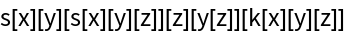Update an expression using only the S combinator transformation rule in a leftmost innermost evaluation scheme:

 In:=Out=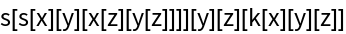### Possible Issues (1)

Sometimes when a replacement is made at a particular position, the other match positions are changed, which prevents all the matches from being activated in a single step:

 In:=Out=